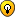## Simple lights effects

Share your awesome Ringo behaviors here!
AngoLito
Posts: 5
Joined: Sat May 21, 2016 8:09 pm

### Simple lights effects

Hi!

I'm starting to "play" with my Ringo; I want to share this simple sketch of light effectsFinally I've copied part of Behavior 5 (color wheel) to change animation's colors.

Is very elemental... but in a dark room Ringo makes a nice effect, specially "moving light"`/* First steps with LedsProgram based on Plumggek guide examplesAngoLito - May 2016 - LuandaEfects:- Moving light- Flash eyes- Dimmer down face LedUsed Behavior 5 sketch (color Wheel) to change color trought Gyro sensor*///Be sure on QUOTATION MARKS pasting code from different Arduino editor fonts can give error#include "RingoHardware.h"      byte i;            //general purpousebyte j=255;     //Bright Led levelbyte k;           //general purpousebyte num_pixel[] = {0,3,4,2,1}; //used to lights Led in order on moving light effectbyte red;       //Red colour valuebyte green;   //Green colour valuebyte blue;     //Blue colour valueunsigned long tiempo;    //Used on <retardo> routine to make delayint presentDirection;int hue;int hueOpposite;double r=0;        //Used in setLedColorHSV routine and dimmer mode (PreLoaded Behavior case 5)double g=0; double b=0;void setup(){  HardwareBegin();          //initialize Ringo's brain to work with his circuitry  PlayStartChirp();           //Play startup chirp and blink eyes  SwitchMotorsToSerial(); //Call "SwitchMotorsToSerial()" before using Serial.print functions as motors & serial share a line  RestartTimer();  NavigationBegin();}    void loop(){    // moving lights  for (k=0; k<19; k++) {     for (i=0; i<5; i++) {    SetPixelRGB(num_pixel[i],red,green,blue);  //set Led    if (num_pixel[i]==4) {      SetPixelRGB( 5, red, green, blue);   //both eyes    }    retardo(9);    OffPixels();    retardo(75);  //Calls subroutine <retardo> for delay and colour adjust  } }  // dimmer down face lights   for (i=0; i<3; i++){                for (j=0; j<255; j++) {                 // dimmer lights from 0 to 255     SetPixelRGB(1,red,green,blue); // lights two down face Leds     SetPixelRGB(2,red,green,blue);     retardo(4);   }     for (j=255; j>0; j--) {                // dimmer lights from 255 to 0     SetPixelRGB(1,red,green,blue);     SetPixelRGB(2,red,green,blue);     retardo(4);    }  } // flashing eyes     j=255;                        //Set maximun bright for Led for (k=0; k<11; k++) {   for (i=0; i<5; i++){    OnEyes(red,green,blue);    retardo(5);    OffPixels();    retardo(65);   }   retardo(375); }} // end of loop() function// In delay time adjust color calling ledwheel()void retardo(int a) {  tiempo = millis();  while (millis()<(tiempo+a)) {    ledwheel();  }}// Calculate color_from Plumgeek PreLoaded Behaviorsvoid ledwheel() {  SimpleGyroNavigation();                          //just does gyroscope (not accelerometer)  presentDirection = abs(GetDegrees());     //gets the present heading in degrees, result is between -180 ~ +180      presentDirection = presentDirection%360;  //If it's been turned multiple times, this divides the large degree number back into a 360 base   hue = presentDirection;                      //Eduardo's function conveniently takes the same 360 degree value we have related to the gyro  hueOpposite = (hue + 180)%360;         //Get the opposite color on the wheel      //SwitchButtonToPixels();                 //User button not used in this sketch    setLedColorHSV(hue,1,1);                 //Calculate the RGB values based on hue}void setLedColorHSV(int h, double s, double v) {  //this is the algorithm to convert from RGB to HSV  double hf=h/60.0;  int i=(int)floor(h/60.0);  //int i=(int)(h/60.0);       //just testing  double f = h/60.0 - i;  double pv = v * (1 - s);  double qv = v * (1 - s*f);  double tv = v * (1 - s * (1 - f));  switch (i)  {  case 0: //rojo dominante    r = v;    g = tv;    b = pv;    break;  case 1: //verde    r = qv;    g = v;    b = pv;    break;  case 2:     r = pv;    g = v;    b = tv;    break;  case 3: //azul    r = pv;    g = qv;    b = v;    break;  case 4:    r = tv;    g = pv;    b = v;    break;  case 5: //rojo    r = v;    g = pv;    b = qv;    break;  }  //set each component to a integer value between 0 and 255  red=constrain((int)j*r,0,255);            //using byte j is a little modification from original code to allows Led dimmer  green=constrain((int)j*g,0,255);  blue=constrain((int)j*b,0,255);} // Ends setLedColorHSV routine`